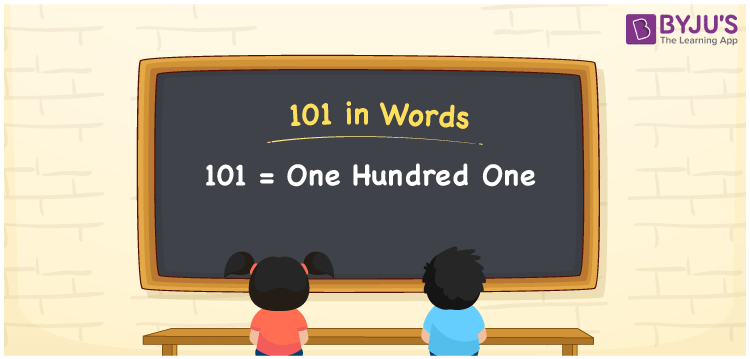# 101 in Words

101 in words is written as “One hundred one”. In Mathematics, 101 is a natural number that is used to represent a value or a quantity. For example, Jack has Rs.101 in his pocket, so he can say, I have One hundred one Rupees in my pocket. Hence, the spelling of 101 is used in different ways in Maths and in real life. Learn how to convert 101 in words here and more about Numbers In Words at BYJU’S.

 101 in Words One hundred one One hundred one in Numbers 101

## 101 in English Words## How to Write 101 in Words?

To write any number in words, we have to check with the position of each digit in the given number. Since, 101 is a three digit number, thus, place value of each digit is given in the below table:

 Hundreds Tens Ones 1 0 1

From the above table, we can see, 1 is at hundreds place, 0 is at tens place and 1 is at ones place. We need to read the number from right to left, in the table. Thus, together we can read the number as One hundred one.

### Expanded Form of 101

We can write the expanded form of 101 as:

1 at Hundreds + 0 at Tens + 1 at Ones

= 1 × 100 + 0 × 10 + 1 × 1

= 101

= One hundred one

101 is a whole number that is succeeded by 100 and preceded by 102. Find more about the number 101 below:

• 101 in Words – One hundred one
• Is 101 an odd number? – Yes
• Is 101 an even number? – No
• Is 101 a perfect square number? – No
• Is 101 a perfect cube number? – No
• Is 101 a prime number? – Yes
• Is 101 a composite number? – No

## Frequently Asked Questions on 101 in words

### What is 101 in words?

101 in words is written as One hundred one.

### What is the rule to write 101 in words?

To write 101 in words, we need to check the position of each digit.

1 → Hundreds

0 → Tens

1 → Ones

Thus the number 101 is read as One hundred one.

### Why 101 is a prime number?

101 is a prime number because it has only two factors, i.e., 1 and 101.